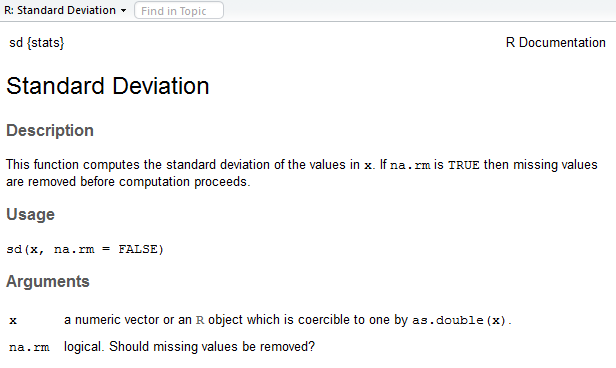# Functions in R Programming

Functions are an important concept in R and we will be using it all the time. In fact, we have already been using functions in our previous examples. For example, we used the summary() to summarize an R object. Similarly, we used the str() function to learn about the structure of an R object.

R functions are objects that evaluate multiple expressions using arguments that are passed to them.

To understand how to use functions, let's take the function to calculate standard deviation in R. The function is defined as sd().

Let's take our vector of stock prices for the past five days. We can use the sd() function to calculate its standard deviation.

#Stock A's Price Data
stock_A <- c(10, 8, 9, 11, 12)
#Calculate Standard Deviation of Stock A
sd(stock_A)


The sd() function takes the vector as an input and returns the standard deviation.

 1.581139


### Function Help

Functions have named arguments which potentially have default values. To learn about how to use a function or its argument list, we can use R documentation. For example, to get help on the sd() function type help(sd) or ?sd. This will open the R documentation with details of the function as shown below:As you can see, the sd() function takes two arguments. The first argument is x which is the vector. The second argument na.rm has a default value of FALSE. This argument checks whether missing values should be removed. Since the argument na.rm has a default value, it is an optional argument which means we don't have to necessarily specify it unless we want to use a value other than the default value.

If you don't want to get full help on the function but just want to get the list of its arguments, you can use the args() function as shown below:

> args(sd)
function (x, na.rm = FALSE)
NULL
>


### Argument Matching

R functions arguments can be matched positionally or by name. So, the following calls to sd() are all equivalent:

#Stock A's Price Data
stock_data <- c(10, 8, 9, 11, 12)
#Calculate Standard Deviation of the Stock
sd(stock_data)
sd(x = stock_data)
sd(x = stock_data, na.rm = FALSE)
sd(na.rm = FALSE, x = stock_data)
sd(na.rm = FALSE, stock_data)


Let's say our dataset contained a missing value. In that case, we would need to specify na.rm = TRUE for the function to evaluate correctly.

> #Stock A's Price Data
> stock_data <- c(10, 8, 9, NA, 11, 12)
>
> #Calculate Standard Deviation of the Stock.
>
> #This will include the 'NA' value in calculation
> #and the function will not evaluate correctly.
> sd(x = stock_data, na.rm = FALSE)
 NA
>
> #This will exclude the 'NA' value in calculation
> #and the function will evaluate correctly.
> sd(x = stock_data, na.rm = TRUE)
 1.581139
>


Most of the time, named arguments are useful on the command line when you have a long argument list and you want to use the defaults for everything except for an argument near the end of the list.

Named arguments also help if you can remember the name of the argument and not its position on the argument list.

## Some More Examples of Functions

Below are some more examples of functions for vectors:

• c() - combines values, vectors, and/or lists to create new objects.
• unique() - returns a vector containing one element for each unique value in the vector.
• rev() - reverse the order of elements in a vector.
• sort() - sorts the elements in a vector.
• append() - append or insert elements in a vector.
• sum() - sum of the elements of a vector.
• min() - minimum value in a vector.
• max() - maximum value in a vector.